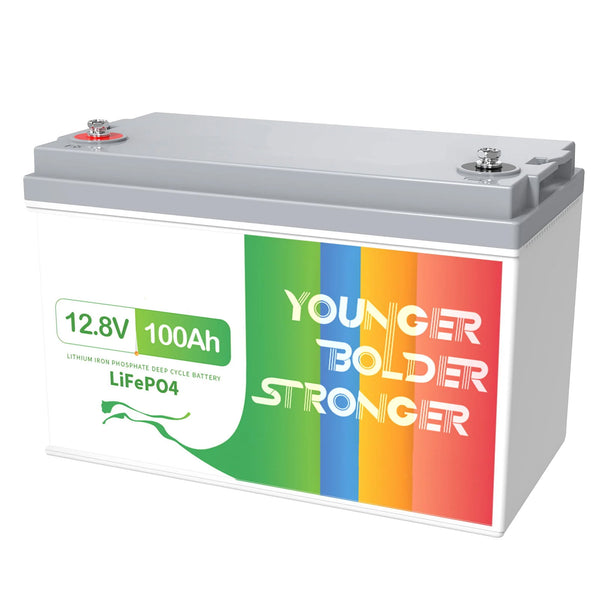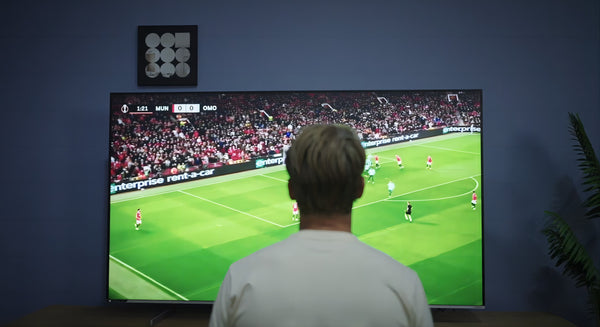# How Long Can a TV Run on a 100Ah Battery?

In activities such as home use and outdoor camping, batteries are often used to power electronic devices. One common question is, how long can a TV run on a 100Ah battery? In this article, we will answer this question and explain the relevant details.

## TV power consumption

Firstly, we need to understand the power consumption of the TV. Different models and sizes of TVs have different power consumption, usually measured in watts (W). Let's assume our TV has a power consumption of 100 watts.## Running time of the TV on a 100Ah battery

Next, we need to calculate the running time of the TV on a 100Ah battery. To do this, we need to convert the battery capacity (measured in amp-hours, Ah) to watt-hours (Wh). This can be done by multiplying the battery capacity by the voltage. Let's assume the battery voltage is 12 volts (V), so the capacity of a 100Ah battery will be 100Ah * 12V = 1200Wh.

Now, we can calculate the running time of the TV on a 100Ah battery. Divide the battery capacity (in watt-hours) by the TV's power consumption, which is 1200Wh / 100W = 12 hours. Therefore, a 100Ah battery can support a TV with a power consumption of 100 watts to run continuously for approximately 12 hours.It is important to note that this calculation result is an estimate based on ideal conditions. In reality, the battery capacity may be affected by other factors such as battery health, ambient temperature, and power consumption of other devices. Therefore, there may be some variations in actual usage.

In summary, a 100Ah battery can support a TV with a power consumption of 100 watts to run continuously for approximately 12 hours. However, this time may vary due to various factors.